Скачать презентацию Area Honors Geometry What is area Think

c20d0500a94d18ecb2e796b471af7040.ppt

• Количество слайдов: 58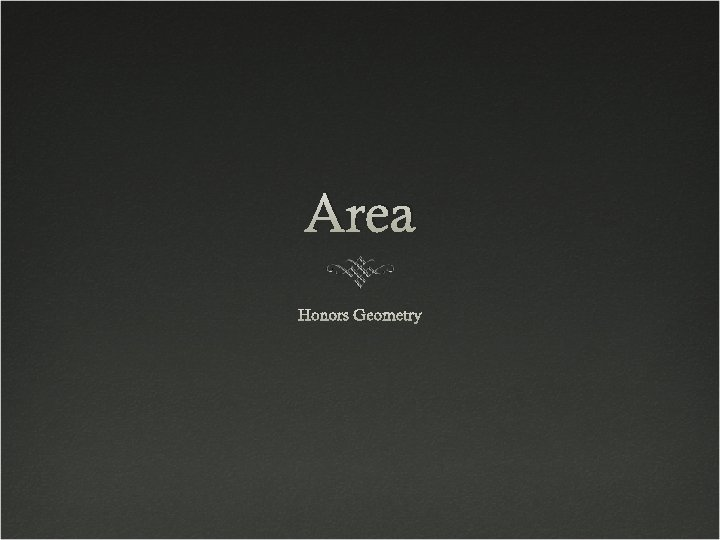Area Honors Geometry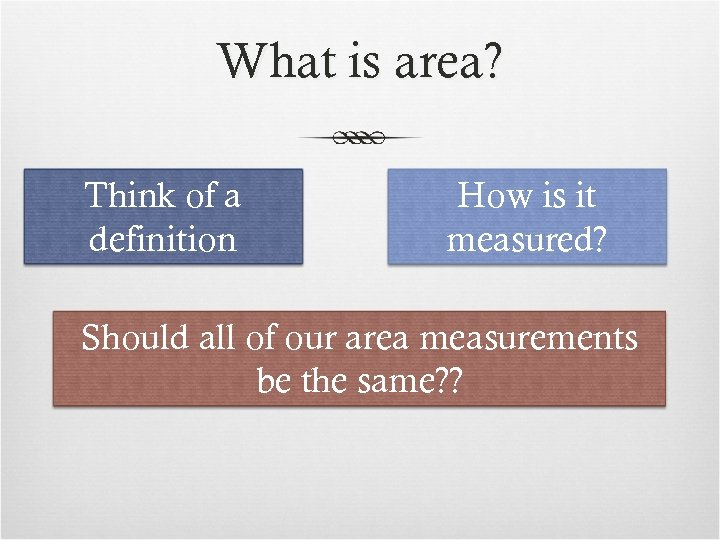What is area? Think of a definition How is it measured? Should all of our area measurements be the same? ?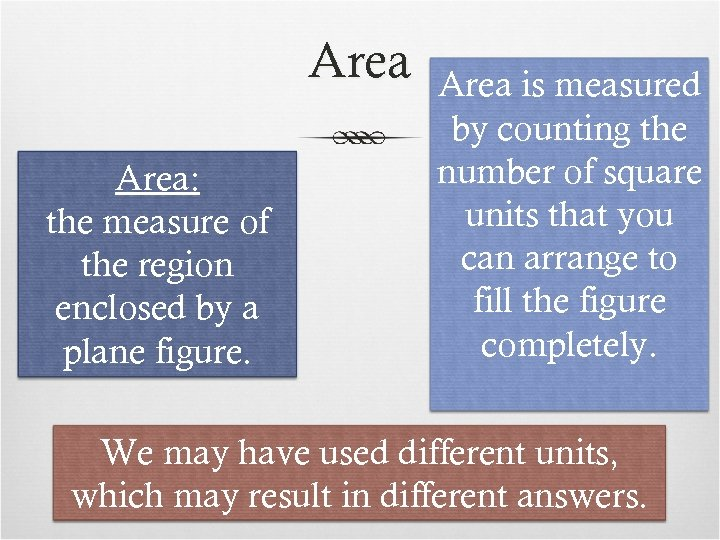Area: the measure of the region enclosed by a plane figure. Area is measured by counting the number of square units that you can arrange to fill the figure completely. We may have used different units, which may result in different answers.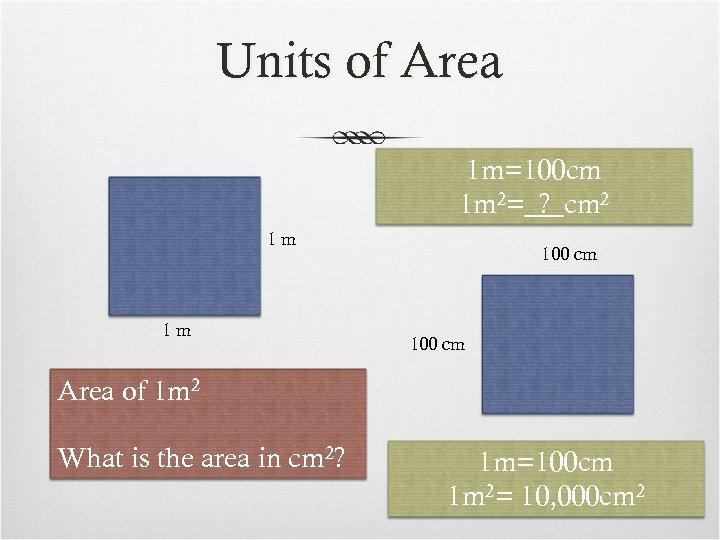Units of Area 1 m=100 cm 1 m 2= ? cm 2 1 m 1 m 100 cm Area of 1 m 2 What is the area in cm 2? 1 m=100 cm 1 m 2= 10, 000 cm 2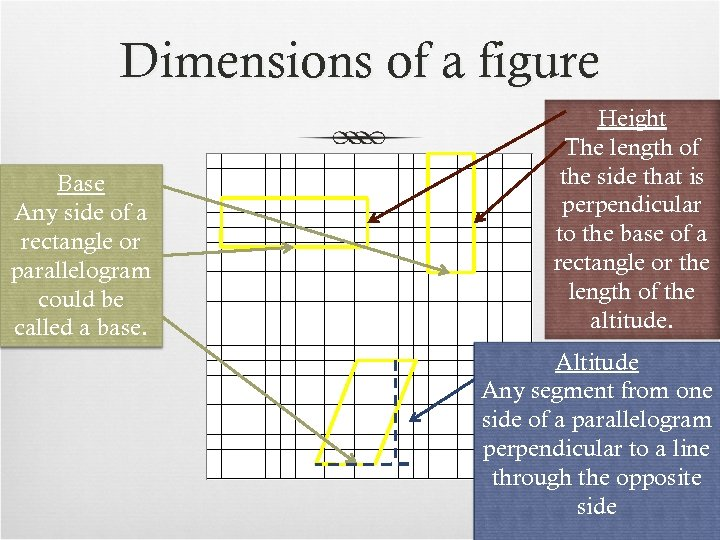Dimensions of a figure Base Any side of a rectangle or parallelogram could be called a base. Height The length of the side that is perpendicular to the base of a rectangle or the length of the altitude. Altitude Any segment from one side of a parallelogram perpendicular to a line through the opposite side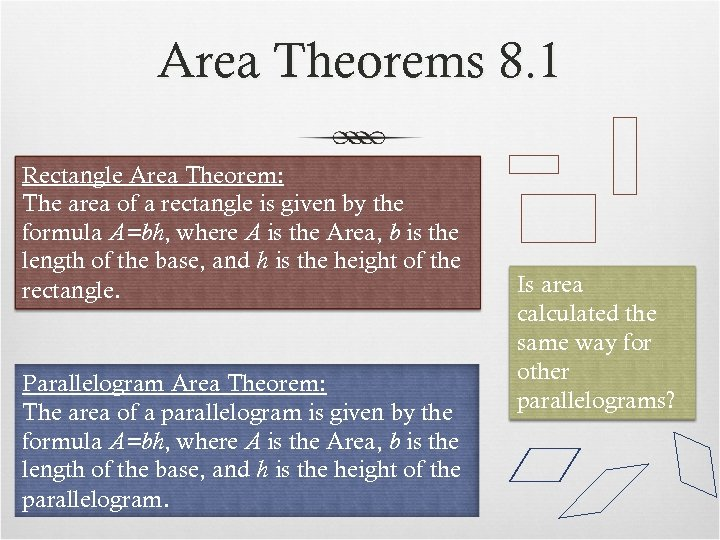Area Theorems 8. 1 Rectangle Area Theorem: The area of a rectangle is given by the formula A=bh, where A is the Area, b is the length of the base, and h is the height of the rectangle. Parallelogram Area Theorem: The area of a parallelogram is given by the formula A=bh, where A is the Area, b is the length of the base, and h is the height of the parallelogram. Is area calculated the same way for other parallelograms?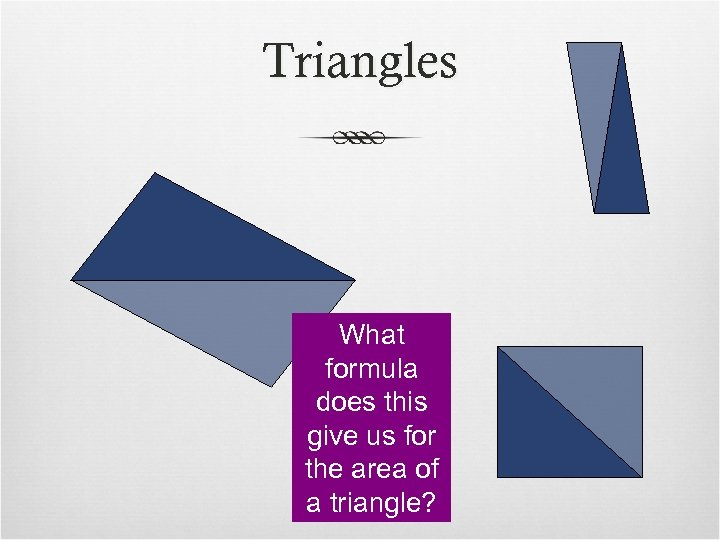Triangles What formula does this give us for the area of a triangle?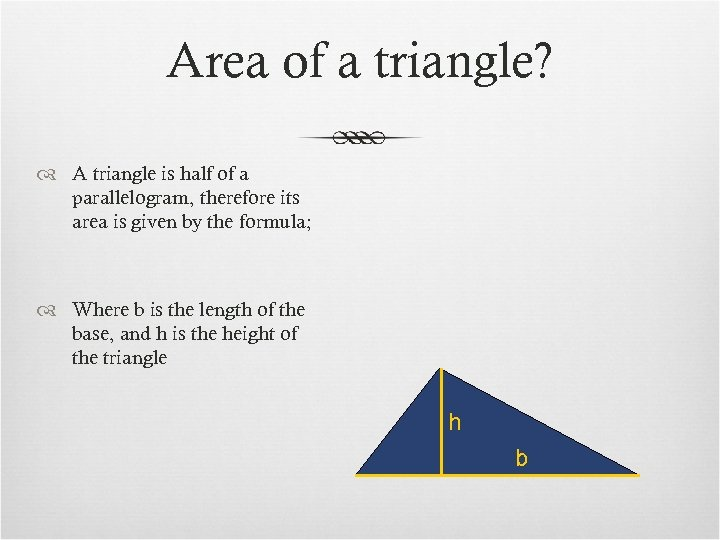Area of a triangle? A triangle is half of a parallelogram, therefore its area is given by the formula; Where b is the length of the base, and h is the height of the triangle h b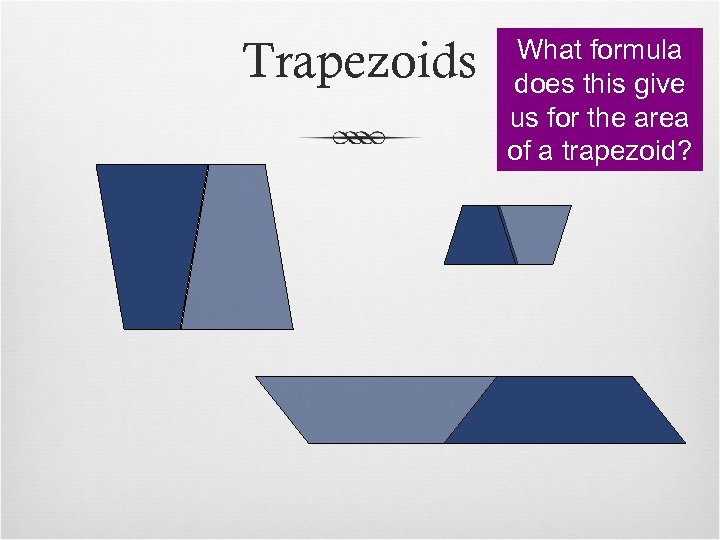Trapezoids What formula does this give us for the area of a trapezoid?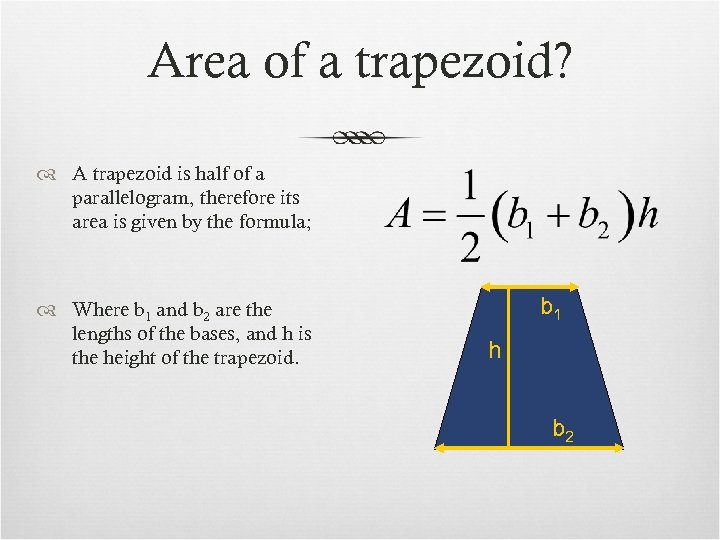Area of a trapezoid? A trapezoid is half of a parallelogram, therefore its area is given by the formula; Where b 1 and b 2 are the lengths of the bases, and h is the height of the trapezoid. b 1 h b 2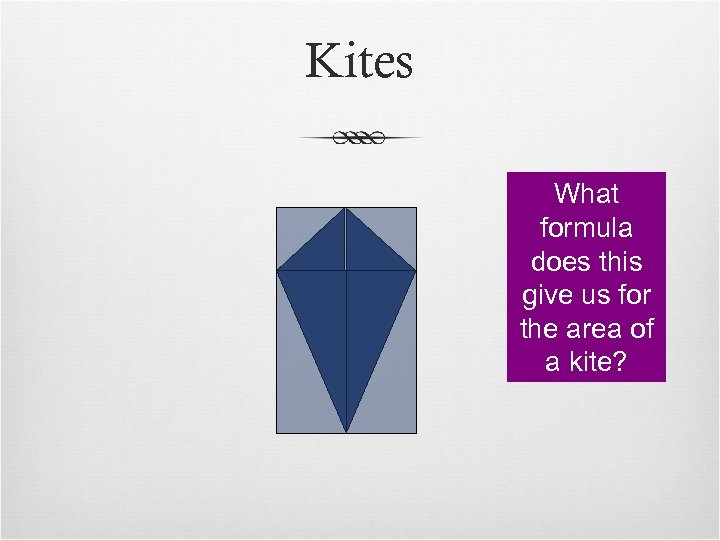Kites What formula does this give us for the area of a kite?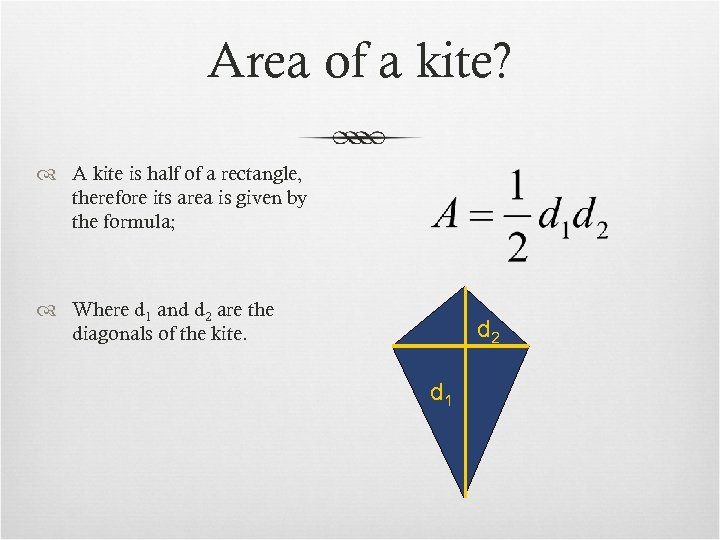Area of a kite? A kite is half of a rectangle, therefore its area is given by the formula; Where d 1 and d 2 are the diagonals of the kite. d 2 d 1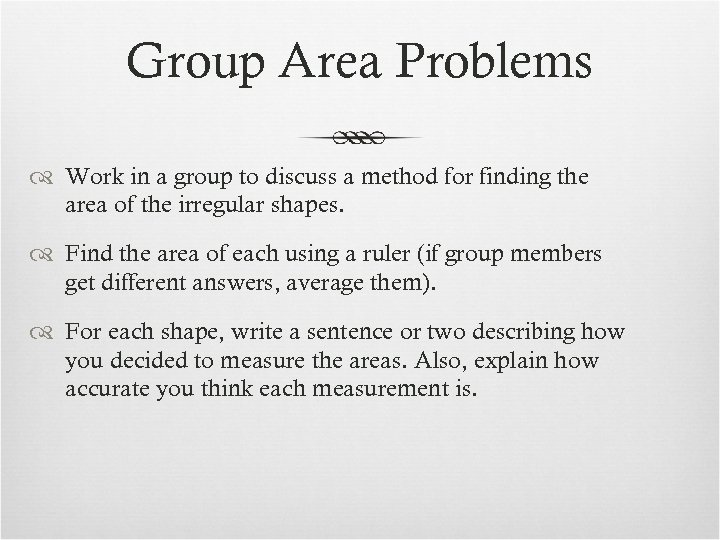Group Area Problems Work in a group to discuss a method for finding the area of the irregular shapes. Find the area of each using a ruler (if group members get different answers, average them). For each shape, write a sentence or two describing how you decided to measure the areas. Also, explain how accurate you think each measurement is.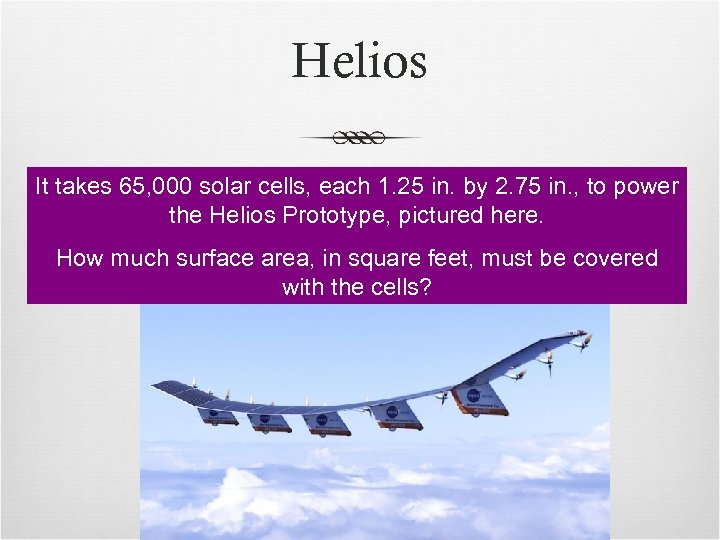Helios It takes 65, 000 solar cells, each 1. 25 in. by 2. 75 in. , to power the Helios Prototype, pictured here. How much surface area, in square feet, must be covered with the cells?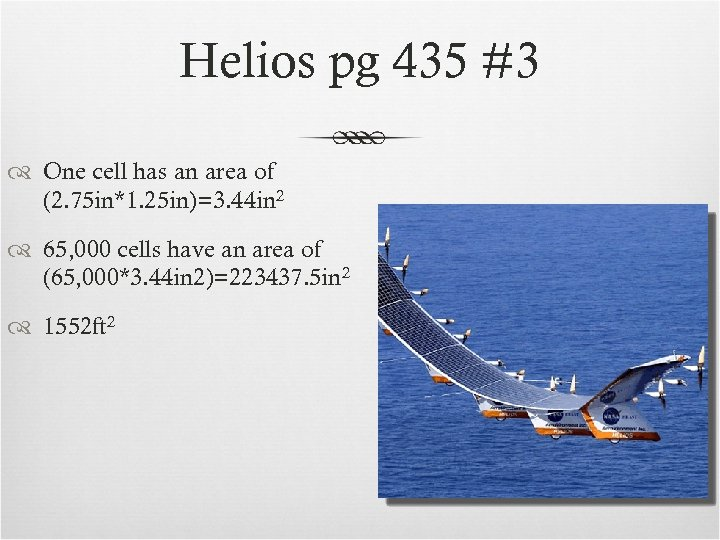Helios pg 435 #3 One cell has an area of (2. 75 in*1. 25 in)=3. 44 in 2 65, 000 cells have an area of (65, 000*3. 44 in 2)=223437. 5 in 2 1552 ft 2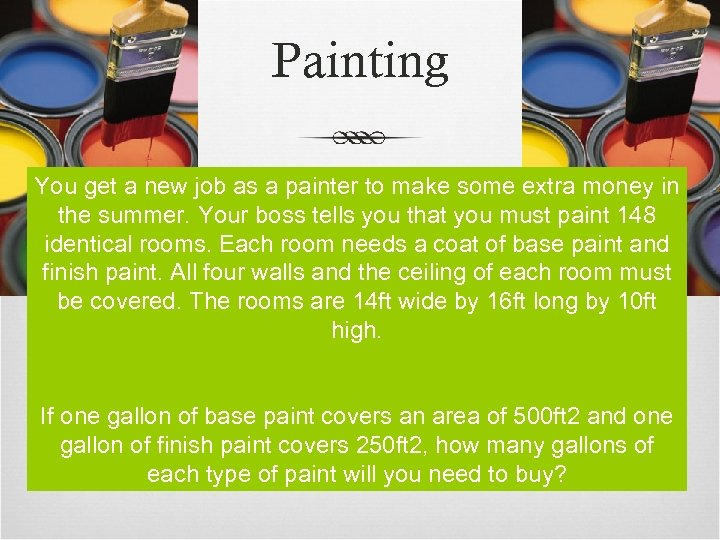Painting You get a new job as a painter to make some extra money in the summer. Your boss tells you that you must paint 148 identical rooms. Each room needs a coat of base paint and finish paint. All four walls and the ceiling of each room must be covered. The rooms are 14 ft wide by 16 ft long by 10 ft high. If one gallon of base paint covers an area of 500 ft 2 and one gallon of finish paint covers 250 ft 2, how many gallons of each type of paint will you need to buy?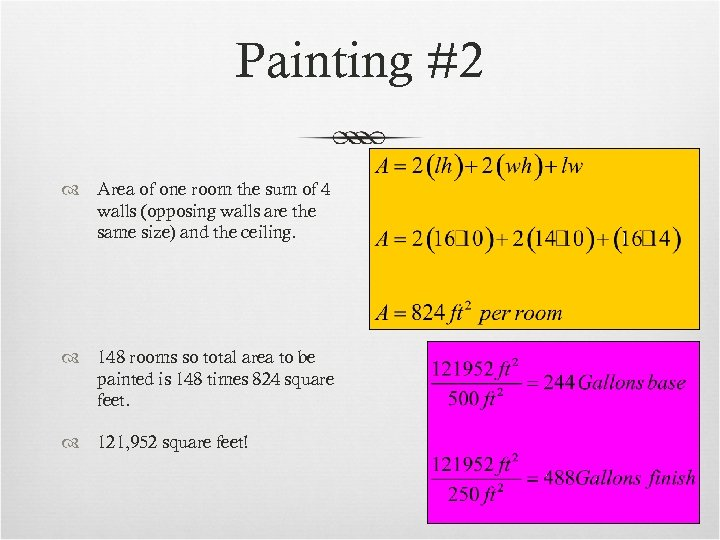Painting #2 Area of one room the sum of 4 walls (opposing walls are the same size) and the ceiling. 148 rooms so total area to be painted is 148 times 824 square feet. 121, 952 square feet!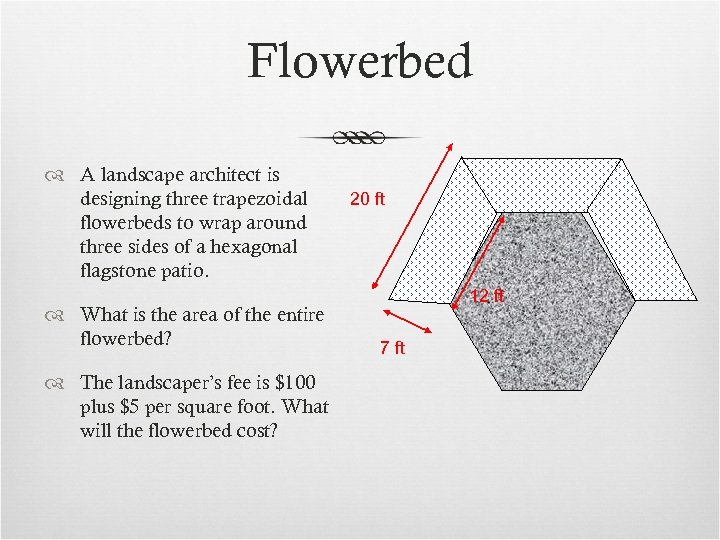Flowerbed A landscape architect is designing three trapezoidal flowerbeds to wrap around three sides of a hexagonal flagstone patio. What is the area of the entire flowerbed? The landscaper’s fee is \$100 plus \$5 per square foot. What will the flowerbed cost? 20 ft 12 ft 7 ft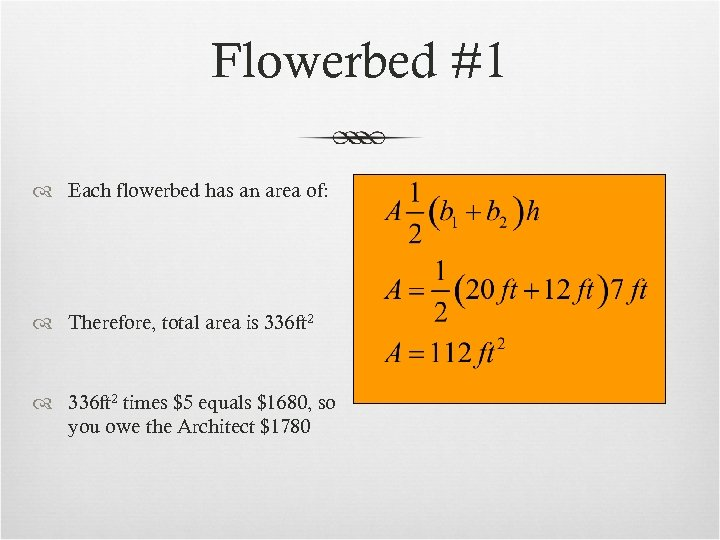Flowerbed #1 Each flowerbed has an area of: Therefore, total area is 336 ft 2 times \$5 equals \$1680, so you owe the Architect \$1780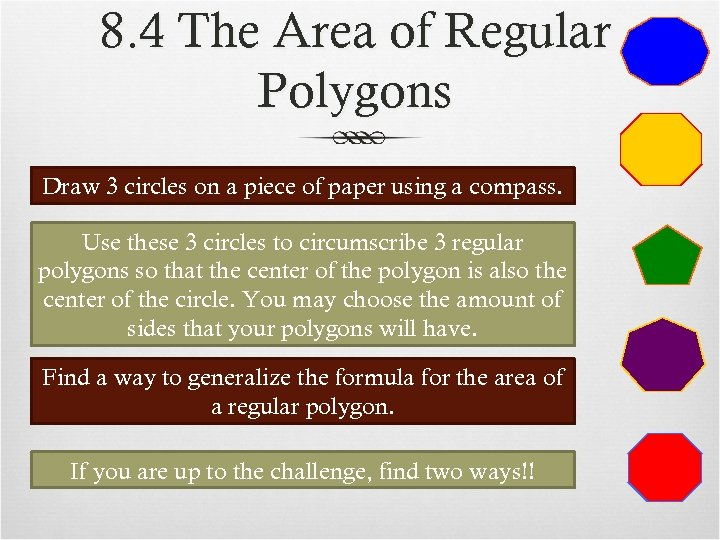8. 4 The Area of Regular Polygons Draw 3 circles on a piece of paper using a compass. Use these 3 circles to circumscribe 3 regular polygons so that the center of the polygon is also the center of the circle. You may choose the amount of sides that your polygons will have. Find a way to generalize the formula for the area of a regular polygon. If you are up to the challenge, find two ways!!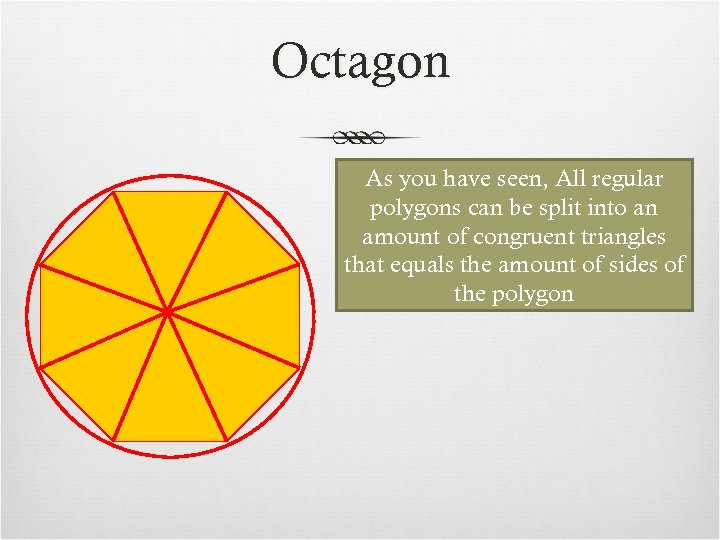Octagon As you have seen, All regular polygons can be split into an amount of congruent triangles that equals the amount of sides of the polygon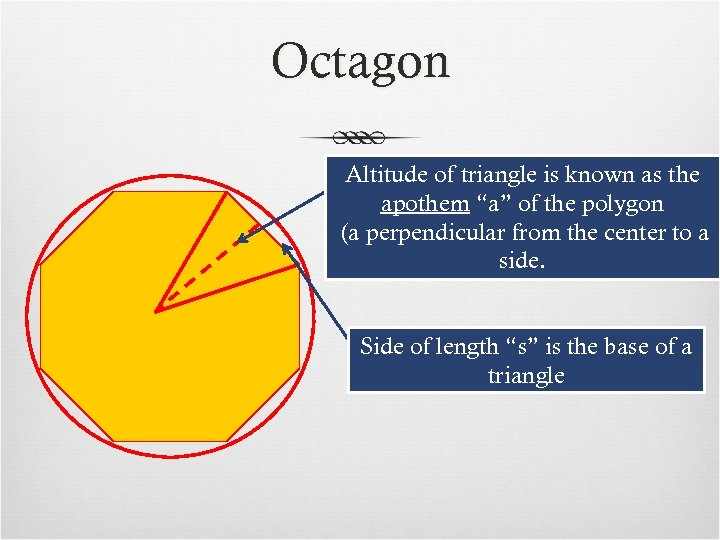Octagon Altitude of triangle is known as the apothem “a” of the polygon (a perpendicular from the center to a side. Side of length “s” is the base of a triangle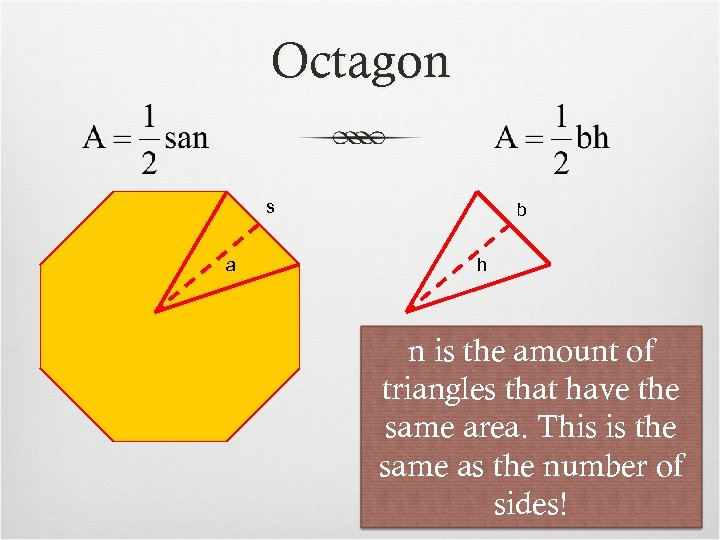Octagon s a b h n is the amount of triangles that have the same area. This is the same as the number of sides!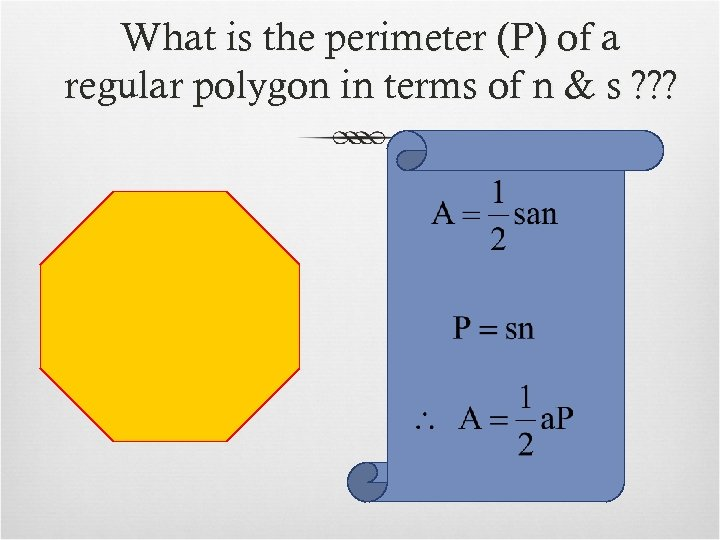What is the perimeter (P) of a regular polygon in terms of n & s ? ? ?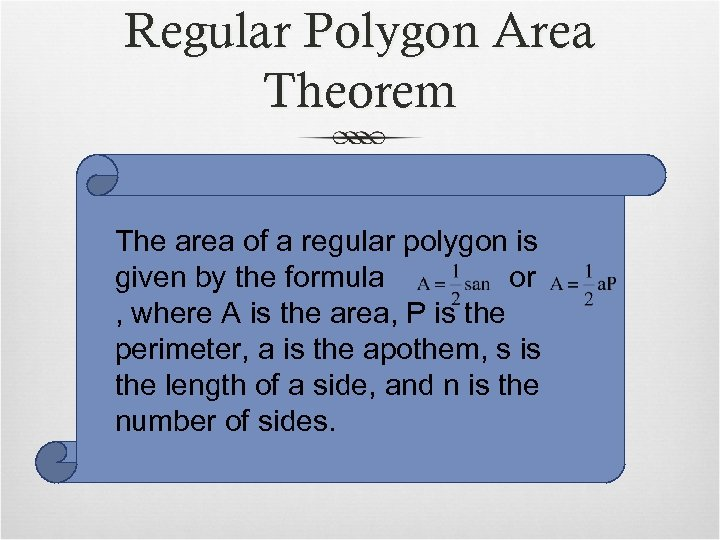Regular Polygon Area Theorem The area of a regular polygon is given by the formula or , where A is the area, P is the perimeter, a is the apothem, s is the length of a side, and n is the number of sides.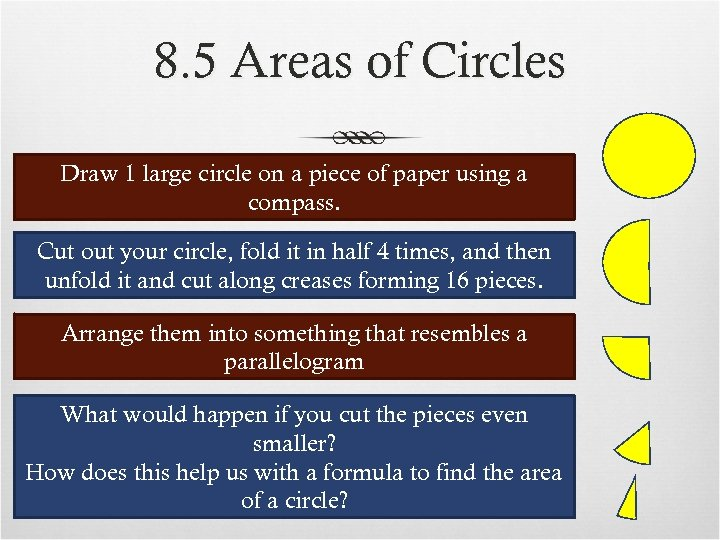8. 5 Areas of Circles Draw 1 large circle on a piece of paper using a compass. Cut out your circle, fold it in half 4 times, and then unfold it and cut along creases forming 16 pieces. Arrange them into something that resembles a parallelogram What would happen if you cut the pieces even smaller? How does this help us with a formula to find the area of a circle?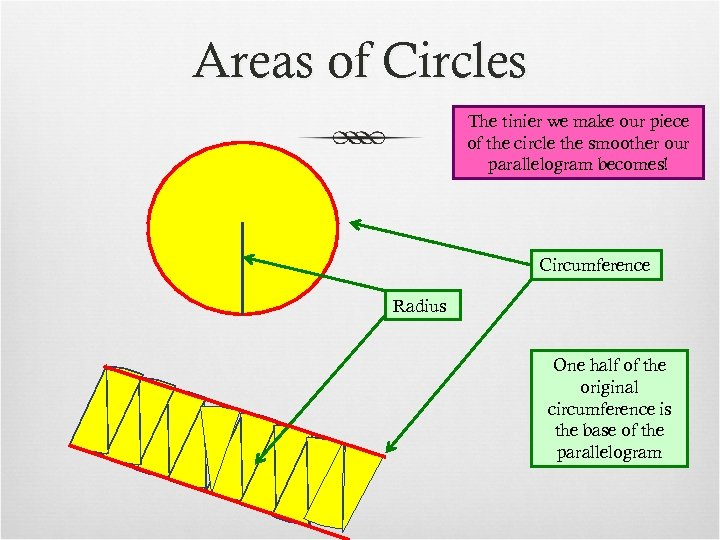Areas of Circles The tinier we make our piece of the circle the smoother our parallelogram becomes! Circumference Radius One half of the original circumference is the base of the parallelogram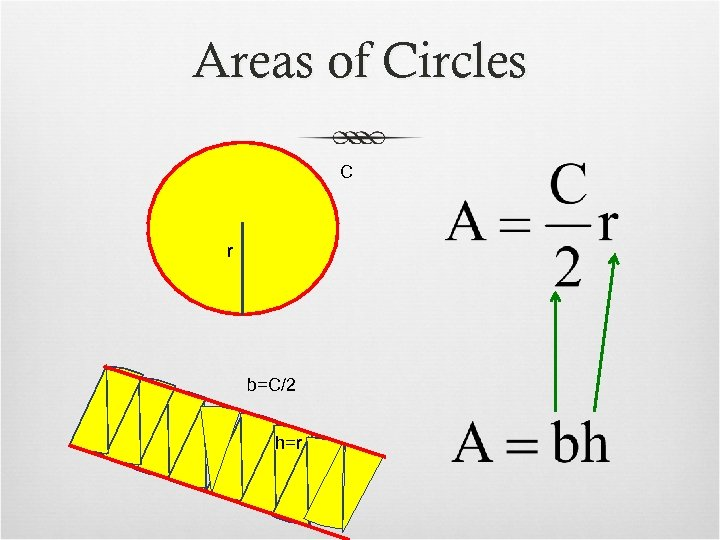Areas of Circles C r b=C/2 h=r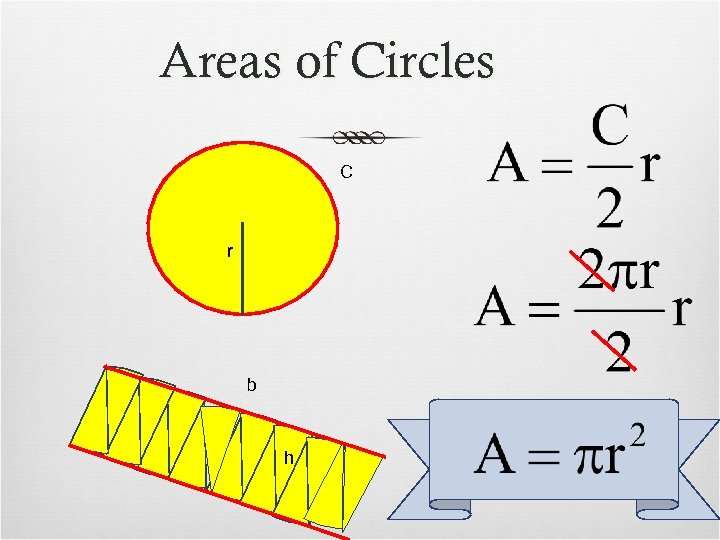Areas of Circles C r b h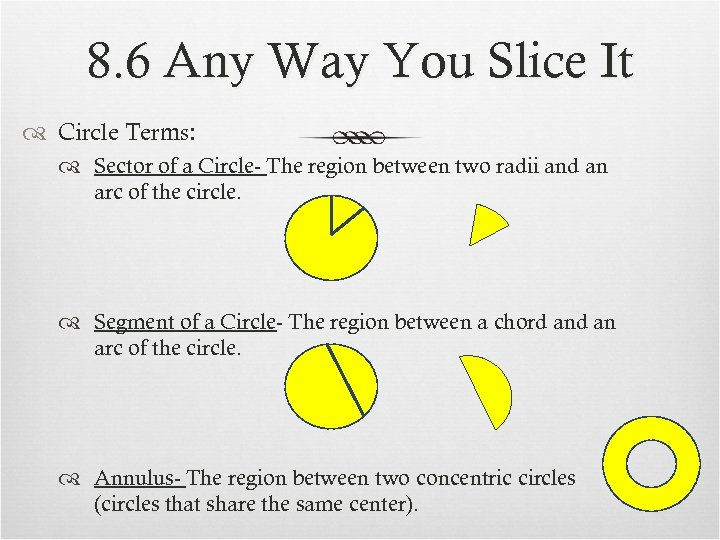8. 6 Any Way You Slice It Circle Terms: Sector of a Circle- The region between two radii and an arc of the circle. Segment of a Circle- The region between a chord an arc of the circle. Annulus- The region between two concentric circles (circles that share the same center).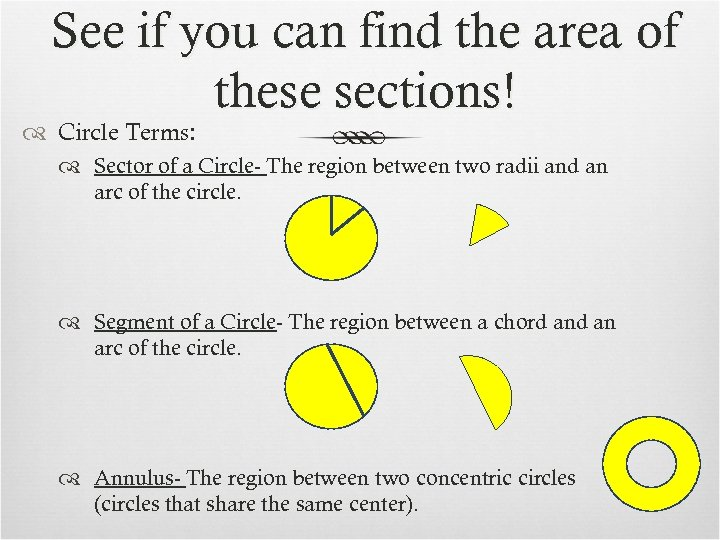See if you can find the area of these sections! Circle Terms: Sector of a Circle- The region between two radii and an arc of the circle. Segment of a Circle- The region between a chord an arc of the circle. Annulus- The region between two concentric circles (circles that share the same center).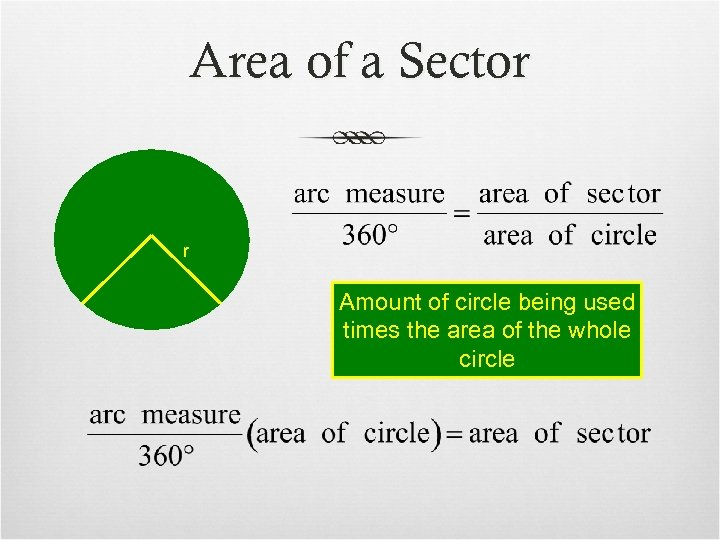Area of a Sector r Amount of circle being used times the area of the whole circle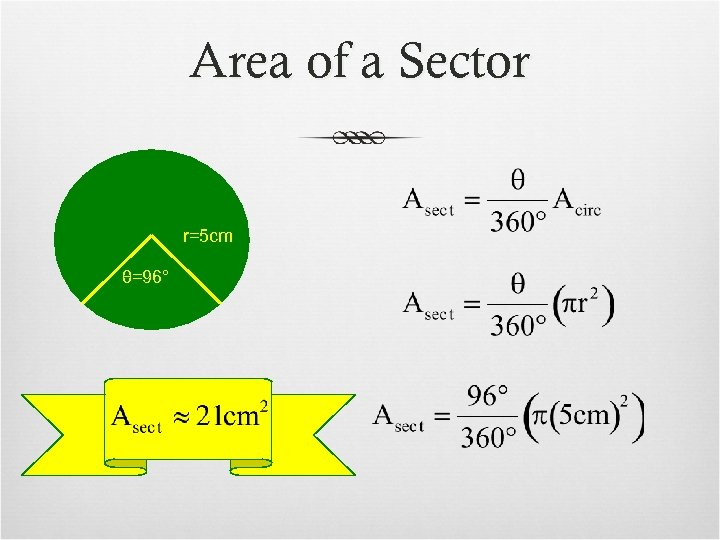Area of a Sector r=5 cm θ=96°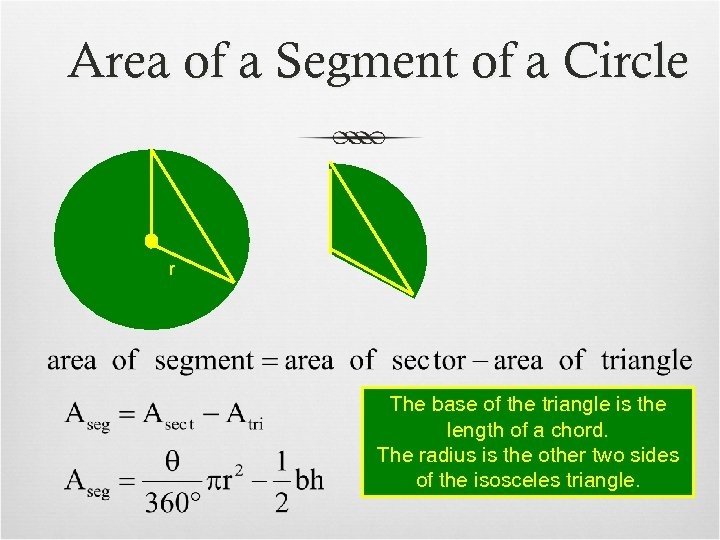Area of a Segment of a Circle r The base of the triangle is the length of a chord. The radius is the other two sides of the isosceles triangle.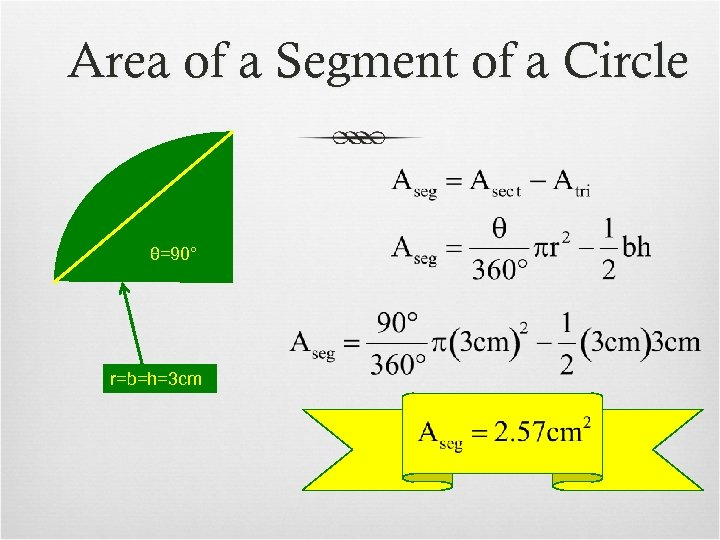Area of a Segment of a Circle θ=90° r=b=h=3 cm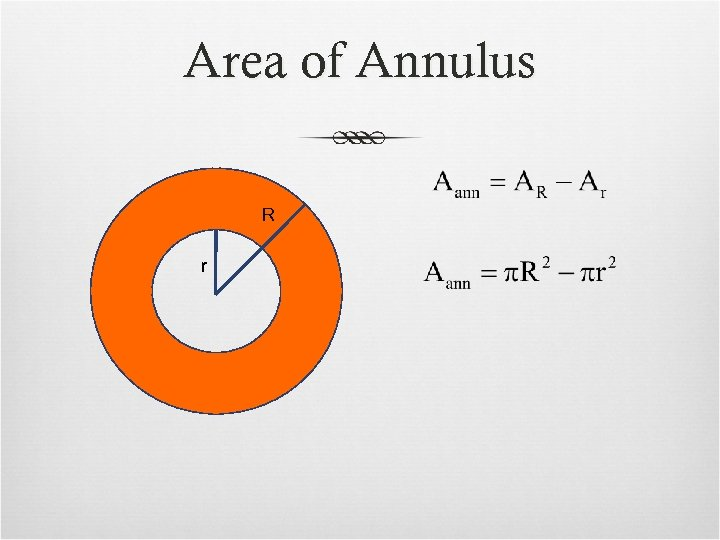Area of Annulus R r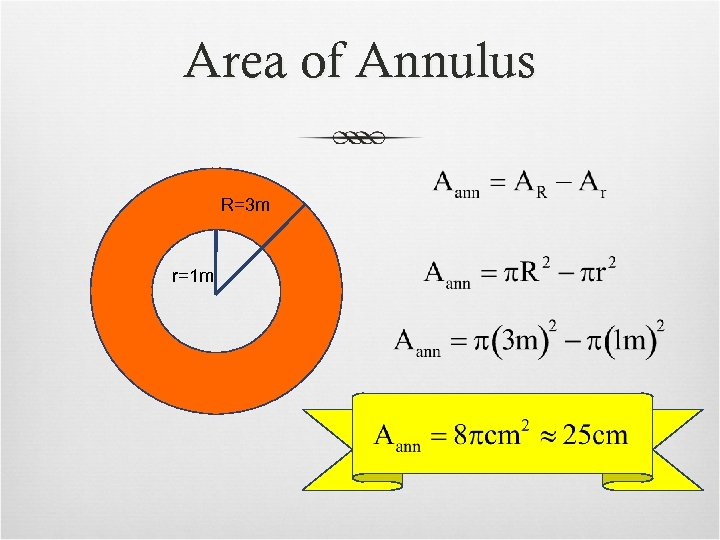Area of Annulus R=3 m r=1 m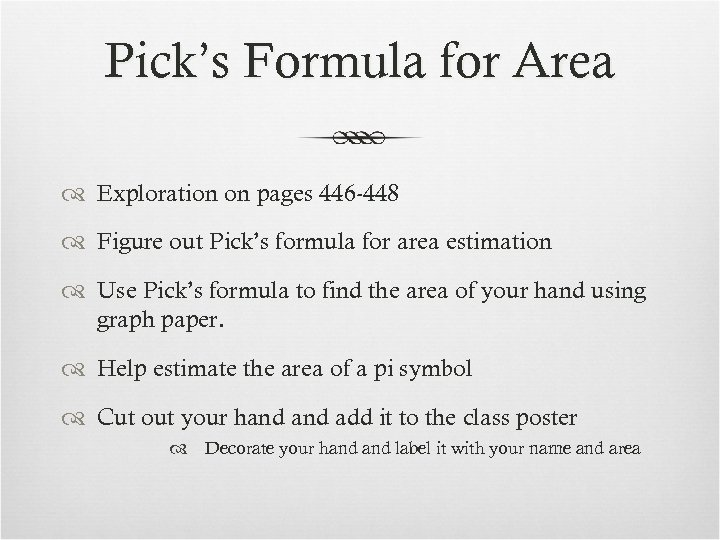Pick’s Formula for Area Exploration on pages 446 -448 Figure out Pick’s formula for area estimation Use Pick’s formula to find the area of your hand using graph paper. Help estimate the area of a pi symbol Cut out your hand add it to the class poster Decorate your hand label it with your name and area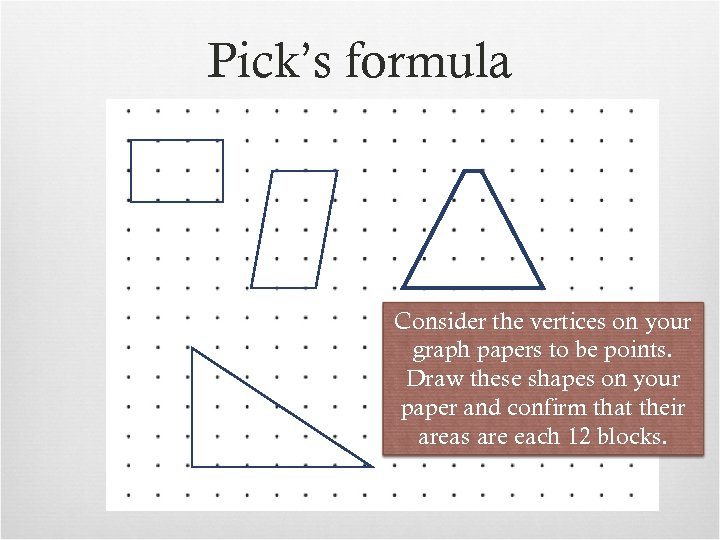Pick’s formula Consider the vertices on your graph papers to be points. Draw these shapes on your paper and confirm that their areas are each 12 blocks.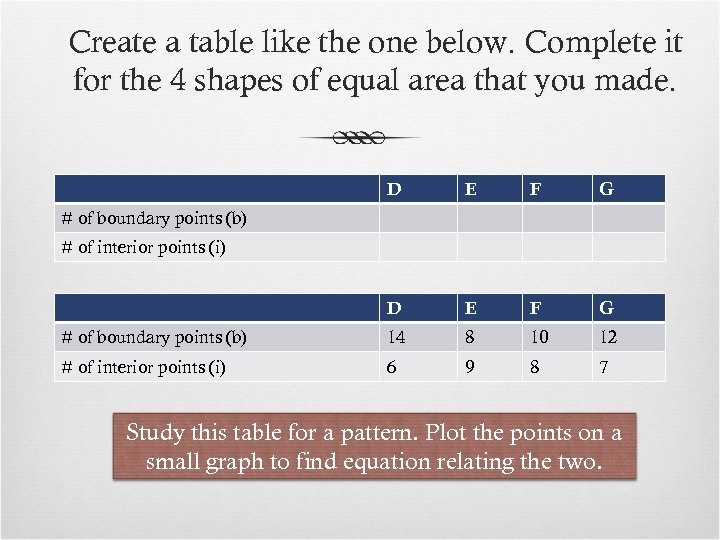Create a table like the one below. Complete it for the 4 shapes of equal area that you made. D E F G # of boundary points (b) 14 8 10 12 # of interior points (i) 6 9 8 7 # of boundary points (b) # of interior points (i) Study this table for a pattern. Plot the points on a small graph to find equation relating the two.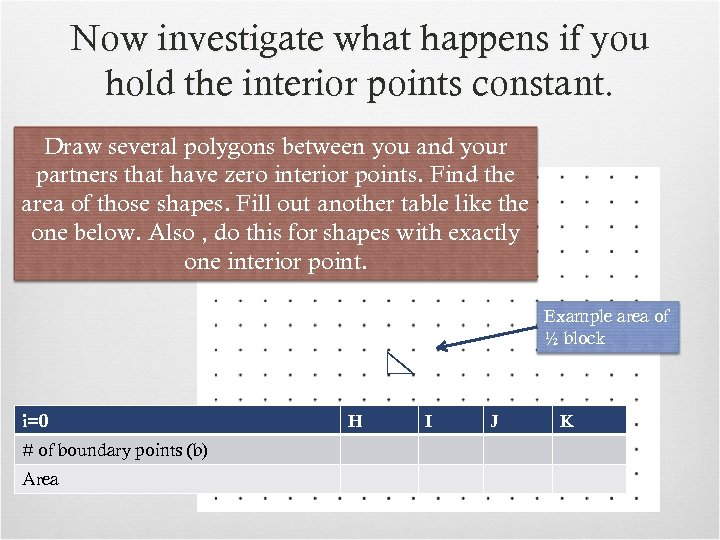Now investigate what happens if you hold the interior points constant. Draw several polygons between you and your partners that have zero interior points. Find the area of those shapes. Fill out another table like the one below. Also , do this for shapes with exactly one interior point. Example area of ½ block i=0 # of boundary points (b) Area H I J K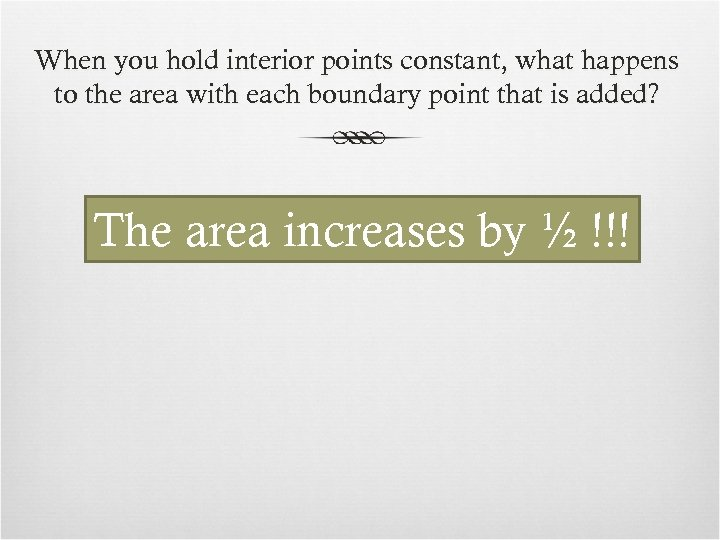When you hold interior points constant, what happens to the area with each boundary point that is added? The area increases by ½ !!!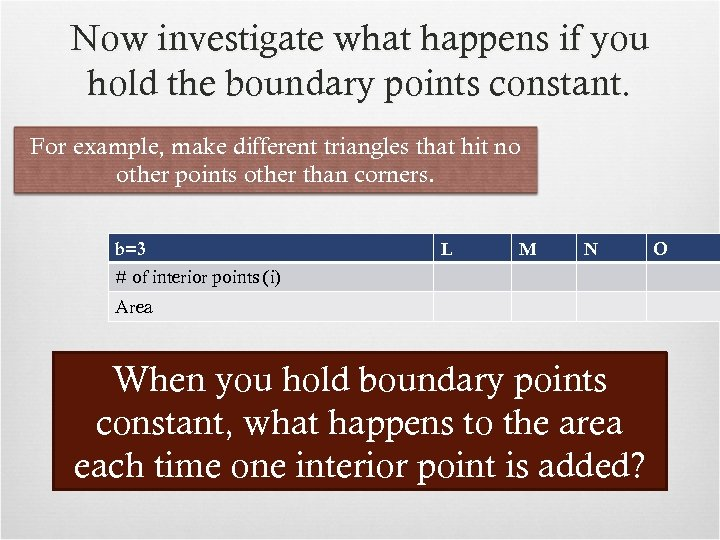Now investigate what happens if you hold the boundary points constant. For example, make different triangles that hit no other points other than corners. b=3 L M N # of interior points (i) Area When you hold boundary points constant, what happens to the area each time one interior point is added? O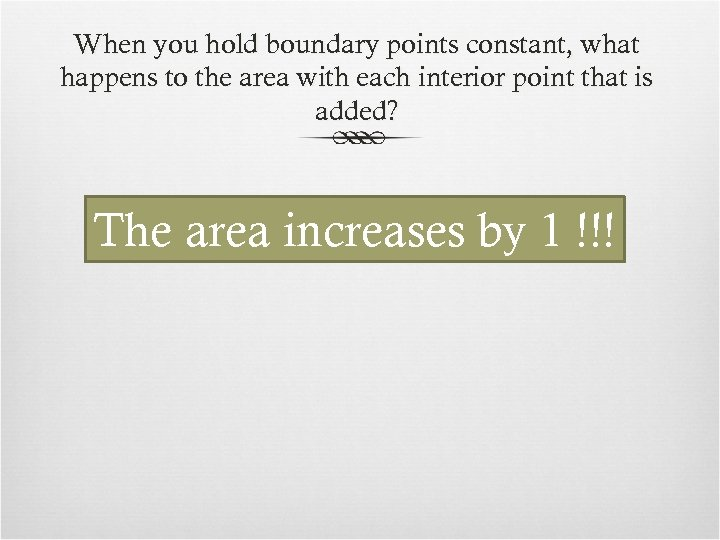When you hold boundary points constant, what happens to the area with each interior point that is added? The area increases by 1 !!!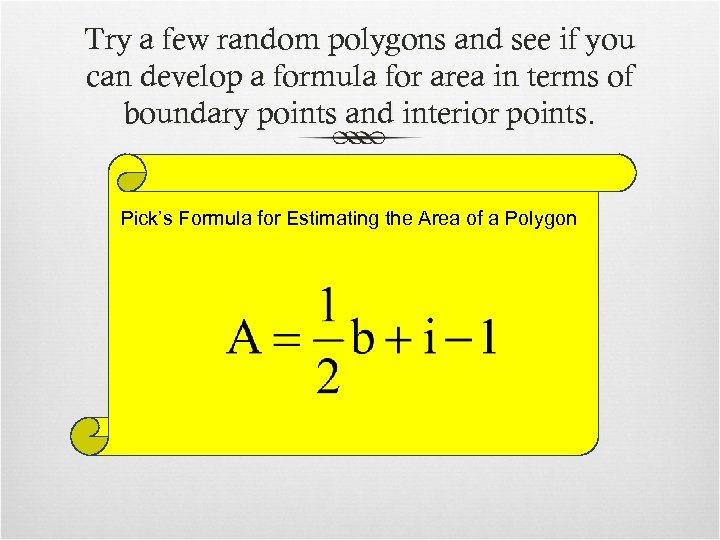Try a few random polygons and see if you can develop a formula for area in terms of boundary points and interior points. Pick’s Formula for Estimating the Area of a Polygon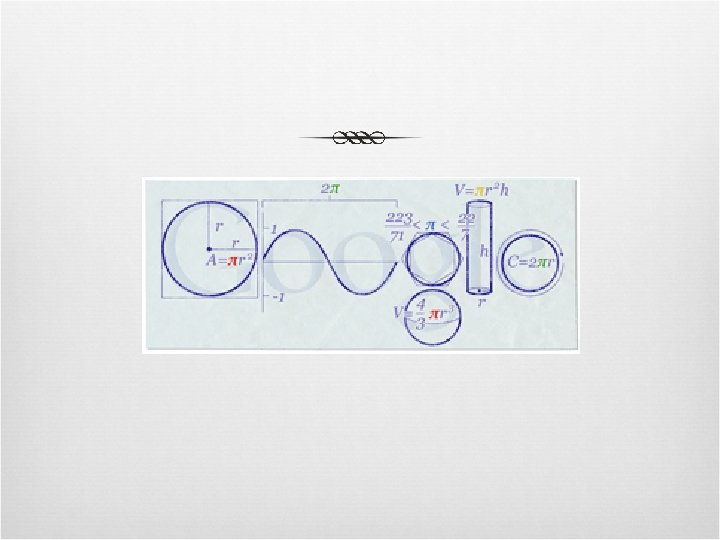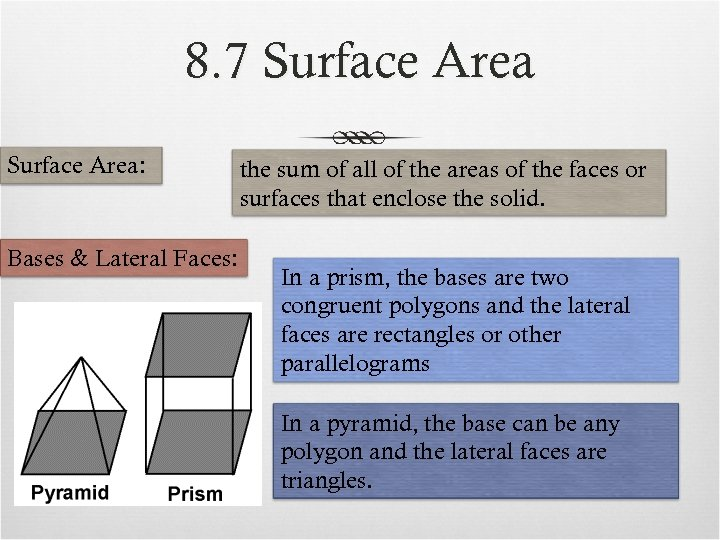8. 7 Surface Area: Bases & Lateral Faces: the sum of all of the areas of the faces or surfaces that enclose the solid. In a prism, the bases are two congruent polygons and the lateral faces are rectangles or other parallelograms In a pyramid, the base can be any polygon and the lateral faces are triangles.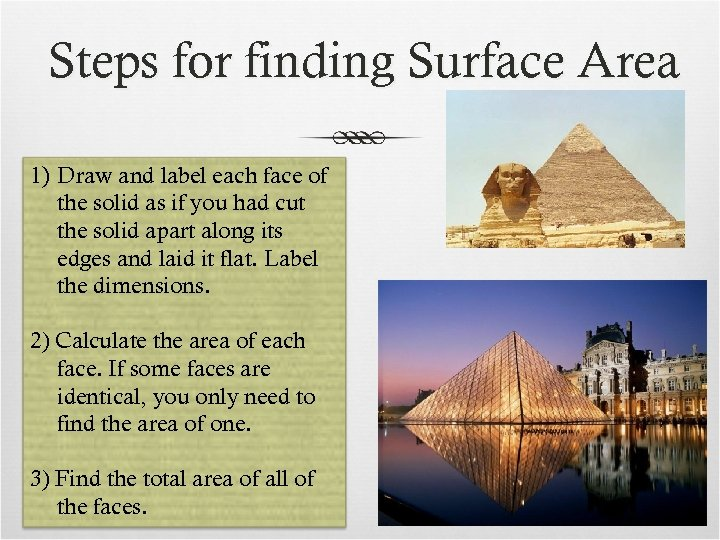Steps for finding Surface Area 1) Draw and label each face of the solid as if you had cut the solid apart along its edges and laid it flat. Label the dimensions. 2) Calculate the area of each face. If some faces are identical, you only need to find the area of one. 3) Find the total area of all of the faces.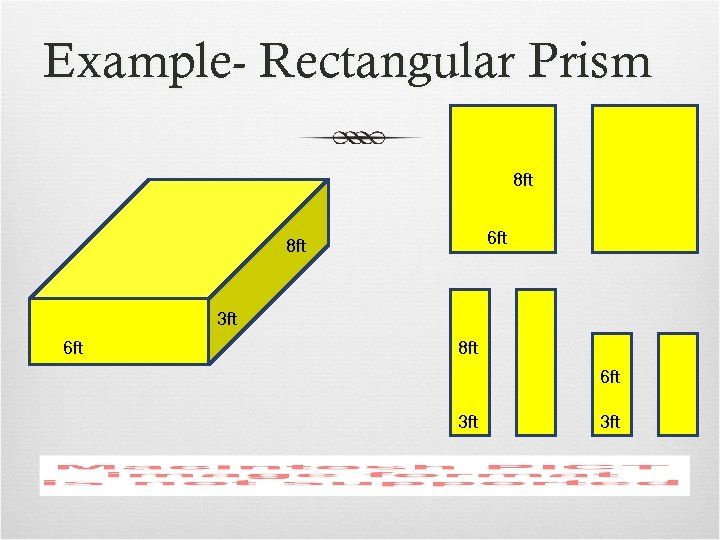Example- Rectangular Prism 8 ft 6 ft 8 ft 3 ft 6 ft 8 ft 6 ft 3 ft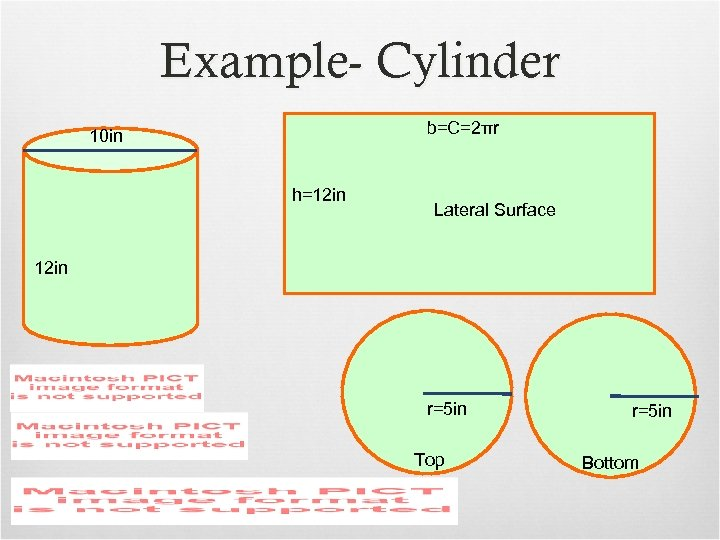Example- Cylinder b=C=2πr 10 in h=12 in Lateral Surface 12 in r=5 in Top r=5 in Bottom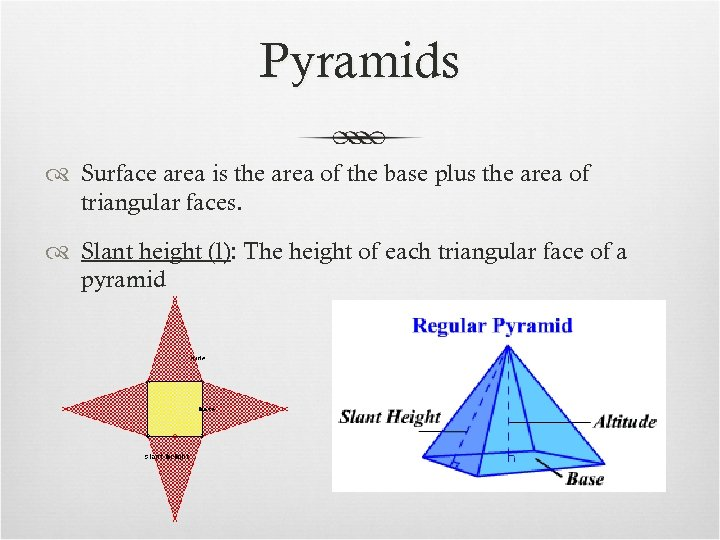Pyramids Surface area is the area of the base plus the area of triangular faces. Slant height (l): The height of each triangular face of a pyramid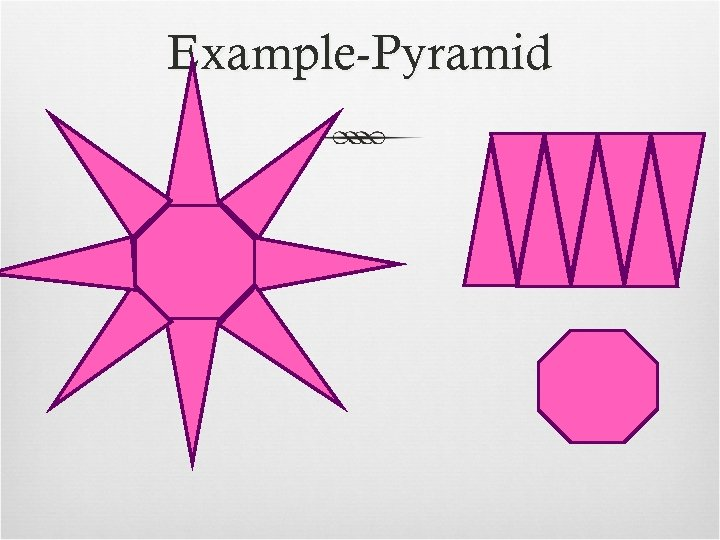Example-Pyramid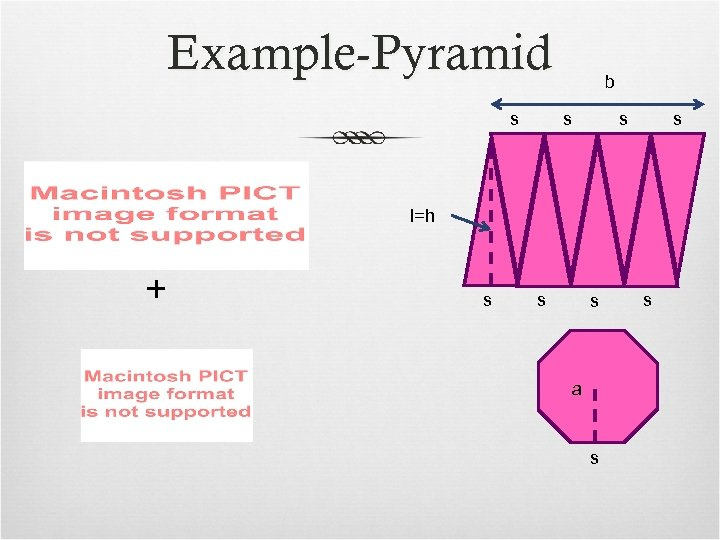Example-Pyramid s b s s s l=h + s s s a s s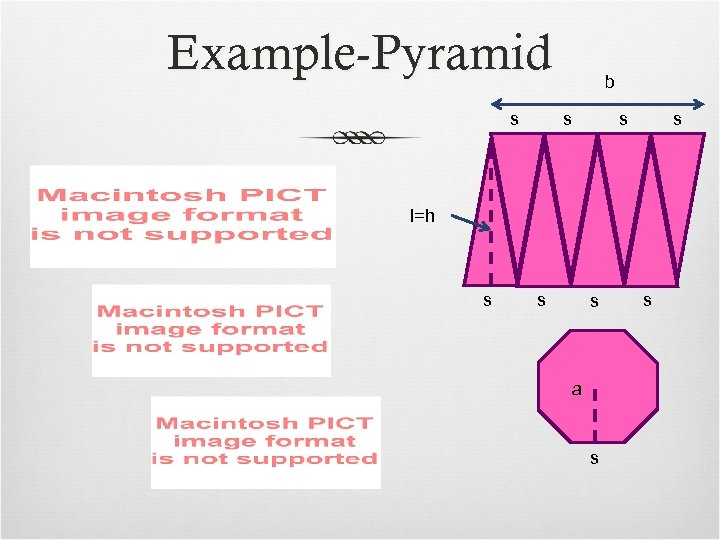Example-Pyramid s b s s s l=h s s s a s s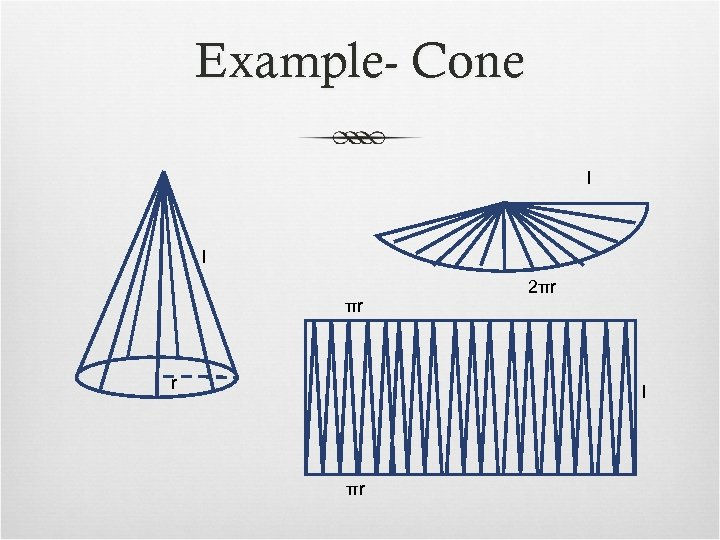Example- Cone l l πr r 2πr l πr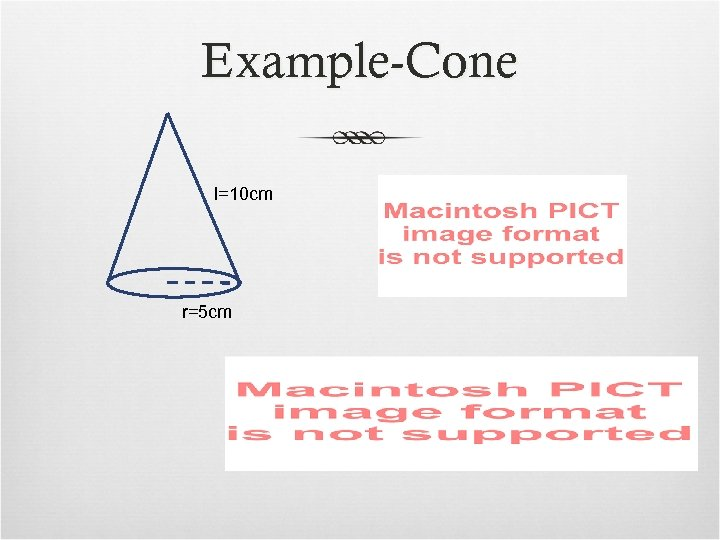Example-Cone l=10 cm r=5 cm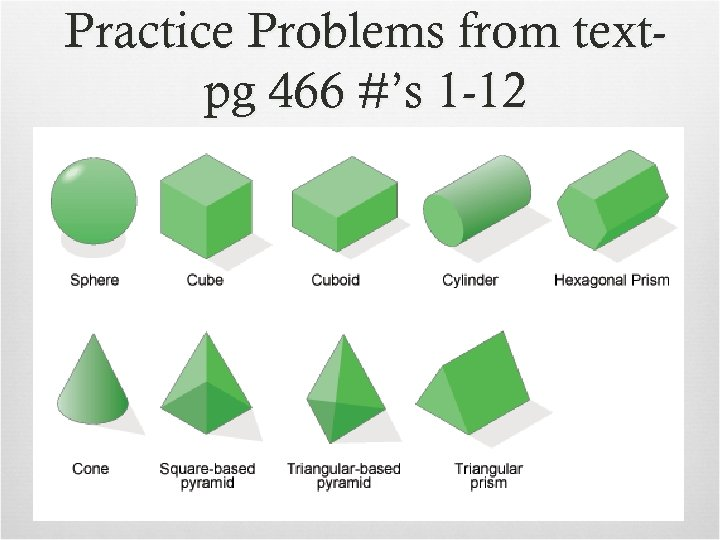Practice Problems from textpg 466 #’s 1 -12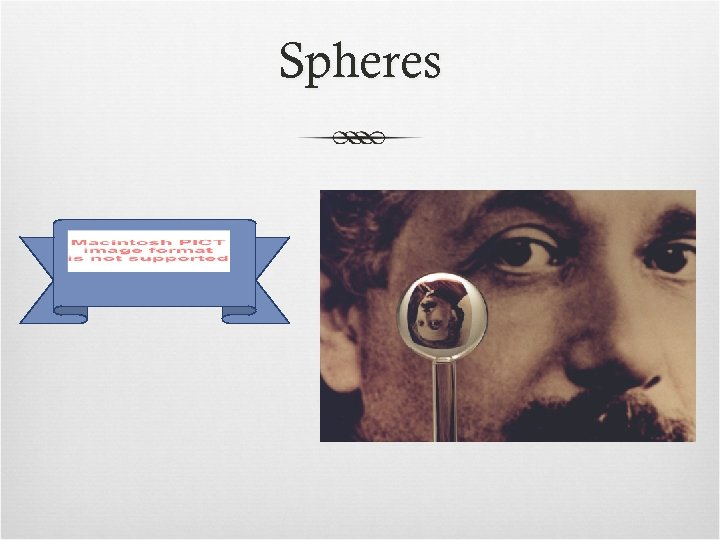Spheres